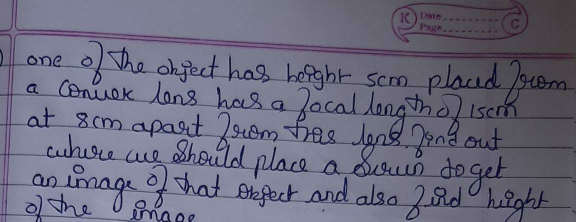"
">

# One of the object has height % cm placed near a convex lens"

Given: f = 15cm, u = -8cm

To find: We have to find the height of the image.

Solution:

We know that,

$\frac{1}{F} \ =\ \frac{1}{v} \ -\ \frac{1}{u}$

$\frac{1}{F} \ +\ \frac{1}{u} =\ \frac{1}{v} \$

$\frac{1}{v} \ =\ \frac{1}{15} \ -\ \frac{1}{8}$ $=\ \frac{-7}{120} \ cm$

$v\ =\ \frac{-120}{7}$, '-' sign indicates that, image is virtual.

A virtual image cannot be caught on a screen

Magnification $M\ =\ \frac{v}{u} \ =\ \frac{\frac{-120}{7}}{-8} \ =\ \frac{15}{7}$

Therefore, $\frac{h^{'}}{h} \ =\ \frac{15}{7} \ \Longrightarrow \ h^{'} \ =\ h\times \ \frac{15}{7}$

Therefore, height of the image $h^{'} \ =\ h\times \ \frac{15}{7}$ = $\ 5\ \times \ \frac{15}{7} \ =\ \frac{75}{7} \ cm$.

Updated on: 10-Oct-2022

27 Views